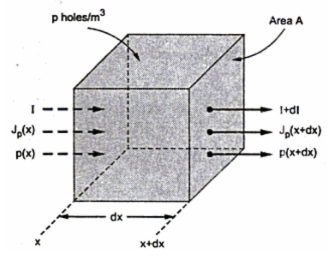### Continuity Equation

The carrier concentration in the body of a semiconductor is a function of time and distance. Mathematically, a practical differential equation governs this functional relationship between carrier concentration, time and distance. Such an equation is called continuity equation. The equation is based on the fact that charge can neither be created nor destroyed.
Consider the infinitesimal n type element of volume of area A and length dx as shown in the Fig. 1. The average hole concentration is p/m3. The current entering the volume at x is I and leaving at x +dx is I + dI. This change in current is because of diffusion. Now due to diffusion the concentration of charge carriers decreases exponentially with the distance.Fig. 1 Conservation of charges
Hence,
...    dI = Number of coulombs per second decreased within the volume             ... (1)
Now if τp is the mean life time of the holes then,
P/τp = Holes per second lost by recombination per unit volume
Due to recombination, number of coulombs per second decreased within the given volume is,
While let g is the rate at which electron hole pairs are generated by thermal generation per unit volume. Due to this, number of coulombs per second increases with the volume
Thus the total change in number of coulombs per second is because of three factors as indicated by the equations (1), (2) and (3).
Total change in holes per unit volume per second is dp/dt. Hence the total change in coulombs per second within the given volume
According to law of conservation of charges,
Note : The negative sign indicates decrease while positive indicates increase in number of coulombs per second.
But J = I/A = Current density
...            I = JA    i.e. dI = AdI   as A is constant.
The total current density J is due to drift and diffusion current.
where    E = Electric field intensity within the volume
If the semiconductor is in thermal equilibrium and subjected to no external electric field then hole density will attain a constant value Po. Under this condition I = 0  i.e. J = 0 and dp/dt = 0 due to equilibrium. Using in equation (7),
o = - (pop)+ g - o
...                 g = pop                                        ........................... (9)
The equation (9) indicates the thermal equilibrium i.e. the rate at which holes are thermally generated just equal to the rate at which holes are lost due to the recombination.
Using equation (8) and equation (9) in equation (7),
This called equation of conservation of charge or the continuity equation.
As holes in n type material are considered, Let us see the suffix n. And as concentration is a function of both time t and distance x, let us use partial differentiation. Hence the final continuity equation takes the form as,
Similarly the continuity equation for the electrons in p-type material can be written as,
Let us discuss the special cases for continuity equation under the condition of zero electric field.
1.1 Concentration independent of Distance and E = 0
As concentration is not dependent on x and E =0, the last two terms on right hand side of the continuity equation becomes zero. Hence equation reduces as,
This is a differential equation interms of pn and the solution of this equation is,

Now τp is mean life time but also time constant of the above equation.
Note : Thus can be defined as the time taken by the injected concentration to fall to 36.78 % of its initial value.
1.2 Concentration Independent of Time and E = 0
As concentration is not dependent on t and E = 0, the left hand side of continuity equation is zero and last term on right hand side is zero.
But    √(Dτ) = Lp = Diffusion length of holes
Hence the solution of the equation (14) takes the form,
1.3 Concentration Varies Sinusoidally With Time and E = 0
The injected concentration varies sinusoidally with an angular frequency of. The sinusoidal variation is indicated in phasor form as,

Substituting in continuity equation with E = 0,

For zero frequency (=0), the equation takes the form as obtained for special case discussed (in sec 1.2)
Hence a.c. solution for 0 can be obtained from d.c. solution by replacing Lp by
Note : Thus continuity equation is the fundamental law governing the flow of charge.